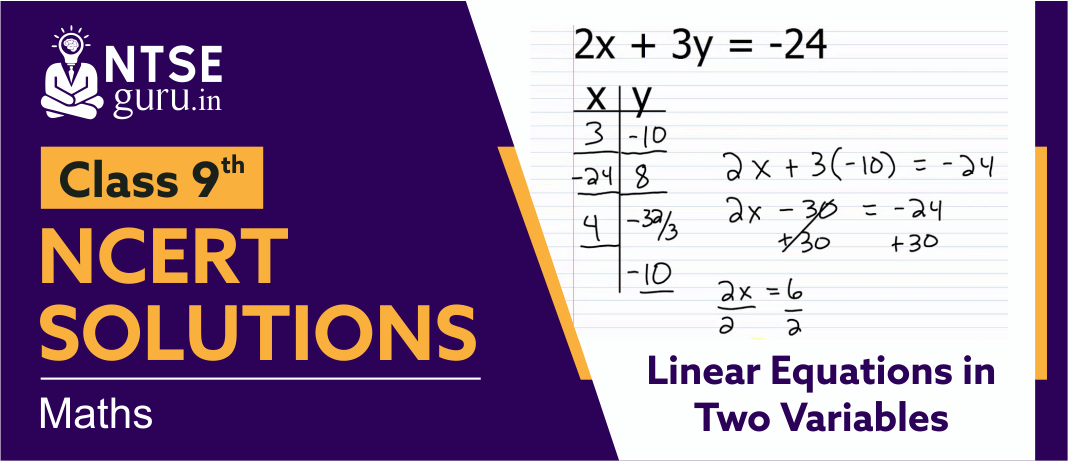# NCERT Solutions For Class 9 Maths Chapter 4NCERT  Solutions for Class 9 Maths Chapter 4, ‘Linear Equations in Two variables’ introduces students with topics such as Linear Equations, graphical presentation of linear equations in two variables & Equations of Lines Parallel to the x-axis and y-axis.

## Linear Equations in Two Variables   -  Class 9 NCERT Solutions

Linear equations are an important tool in science and many everyday applications. It allows scientists to describe relationships between two variables in the physical world, make predictions, calculate rates, and make conversions. Graphing linear equations helps make trends visible. Given below solutions on ‘Linear Equations in Two Variables   -  Class 9 NCERT’ will help students in relating more with the topic.

In addition to the above solutions, students are also advised to follow sample papers, previous years solved papers, and practice sets. All this material can be accessed from our website or from our free mobile app NTSE Guru.